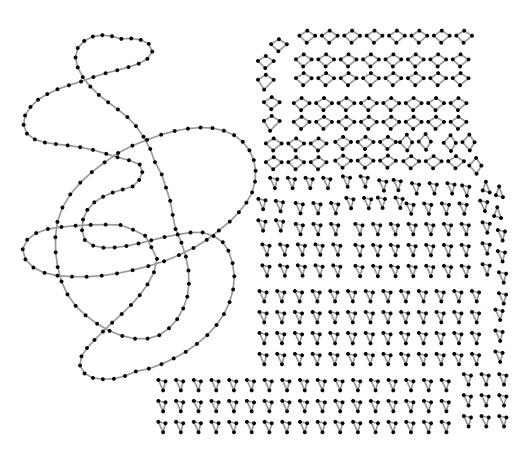# Exactly solvable random graph ensemble with extensively many short cycles

Controlling analytically second or higher-order properties of networks is a great mathematical challenge.

Journal of Physics A: Mathematical and Theoretical 51, 85101 (2018)

F. Lopez, P. Barucca, M. Fekom, A. CoolenWe introduce and analyse ensembles of 2-regular random graphs with a tuneable distribution of short cycles. The phenomenology of these graphs depends critically on the scaling of the ensembles' control parameters relative to the number of nodes. A phase diagram is presented, showing a second order phase transition from a connected to a disconnected phase. We study both the canonical formulation, where the size is large but fixed, and the grand canonical formulation, where the size is sampled from a discrete distribution, and show their equivalence in the thermodynamical limit. We also compute analytically the spectral density, which consists of a discrete set of isolated eigenvalues, representing short cycles, and a continuous part, representing cycles of diverging size.